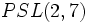# Alternating group:A8

View a complete list of particular groups (this is a very huge list!)[SHOW MORE]

## Definition

This group is defined in the following equivalent ways:

1. It is the alternating group of degree eight, i.e., over a set of size eight.
2. It is the projective special linear group of degree four over the field of two elements, i.e.,$PSL(4,2)$. It is also the special linear group$SL(4,2)$, the projective general linear group$PGL(4,2)$, and the general linear group$GL(4,2)$.

This is one member of the smallest order pair of non-isomorphic finite simple non-abelian groups having the same order. The other member of this pair is projective special linear group:PSL(3,4).

### Equivalence of definitions

The equivalence between the various definitions within (2) follows from isomorphism between linear groups over field:F2.

## Arithmetic functions

Want to compare and contrast arithmetic function values with other groups of the same order? Check out groups of order 20160#Arithmetic functions

### Basic arithmetic functions

Function Value Similar groups Explanation
order (number of elements, equivalently, cardinality or size of underlying set) 20160 groups with same order As alternating group$A_n, n = 8$:$n!/2 = 8!/2 = (8 \cdot 7 \cdot 6 \cdot 5 \cdot 4 \cdot 2 \cdot 3 \cdot 1)/2 = 20160$
As general linear group$GL(4,q), q = 2$:$(q^4 - 1)(q^4 - q)(q^4 - q^2)(q^4 - q^3) = (2^4 - 1)(2^4 - 2)(2^4 - 2^2)(2^4 - 2^3) = 15 \cdot 14 \cdot 12 \cdot 8 = 20160$
exponent of a group 420 groups with same order and exponent of a group | groups with same exponent of a group As$A_n, n= 8$,$n$ even:$\operatorname{lcm}\{ 1,2,3,\dots,n-1\} = \operatorname{lcm} \{ 1,2,3,\dots,7 \} = 420$
As$GL(4,q), q = 2, p = 2$:$p^2 \operatorname{lcm} \{ q - 1, q^2 - 1, q^3 - 1, q^4 - 1 \} = 4 \operatorname{lcm} \{ 1,3,7,15 \} = 4(105) = 420$
derived length -- -- not a solvable group
nilpotency class -- -- not a nilpotent group
Frattini length 1 groups with same order and Frattini length | groups with same Frattini length Frattini-free group: intersection of all maximal subgroups is trivial
minimum size of generating set 2 groups with same order and minimum size of generating set | groups with same minimum size of generating set

### Arithmetic functions of a counting nature

Function Value Similar groups Explanation for function value
number of conjugacy classes 14 groups with same order and number of conjugacy classes | groups with same number of conjugacy classes As$A_n, n = 8$: (2 * (number of self-conjugate partitions of 8)) + (number of conjugate pairs of non-self-conjugate partitions of 8) =$(2 * 2) + 10 = 14$ (more here)
As$GL(4,q), q = 2$:$q^4 - q = 2^4 - 2 = 14$ (more here)
As$SL(4,q), q = 2$:$q^3 + q^2 + q = 2^3 + 2^2 + 2 = 14$ (more here)
See element structure of alternating group:A8
number of conjugacy classes of subgroups 137 groups with same order and number of conjugacy classes of subgroups | groups with same number of conjugacy classes of subgroups See subgroup structure of alternating group:A8, subgroup structure of alternating groups
number of subgroups 48337 groups with same order and number of subgroups | groups with same number of subgroups See subgroup structure of alternating group:A8, subgroup structure of alternating groups

## Elements

Further information: element structure of alternating group:A8

## Subgroups

Further information: subgroup structure of alternating group:A8

### Quick summary

Item Value
Number of subgroups 48337
Compared with$A_n, n = 3,4,5,\dots$: 2, 10, 59, 501, 3786, 48337, ...
Number of conjugacy classes of subgroups 137
Compared with$A_n, n = 3,4,5,\dots$: 2, 5, 9, 22, 40, 137, ...
Number of automorphism classes of subgroups 112
Compared with$A_n, n = 3,4,5,\dots$: 2, 5, 9, 16, 37, 112, ...
Isomorphism classes of Sylow subgroups 2-Sylow: unitriangular matrix group:UT(4,2) (order 64).
3-Sylow: elementary abelian group:E9 (order 9).
5-Sylow: cyclic group:Z5 (order 5)
7-Sylow: cyclic group:Z7 (order 7)
Hall subgroups Other than the whole group, the trivial subgroup, and the Sylow subgroups, there are$\{ 2,3 \}$-Hall subgroups of order 576.
maximal subgroups maximal subgroups have orders 360, 576, 720, 1344, 2520
normal subgroups only the whole group and the trivial subgroup, because the group is simple. See alternating groups are simple.
subgroups that are simple non-abelian groups (other than the whole group) alternating group:A5 (order 60), projective special linear group:PSL(3,2) (order 168, the embedding arises via the natural permutation representation on the two-dimensional projective space over field:F2, which has size$2^2 + 2 + 1 = 7$, or via embedding it as$PSL(2,7)$ in the alternating group on a set of size$7 + 1 = 8$), alternating group:A6 (order 360), alternating group:A7 (order 2520)

## Linear representation theory

Further information: linear representation theory of alternating group:A8

### Summary

Item Value
degrees of irreducible representations over a splitting field 1,7,14,20,21,21,21,28,35,45,45,56,64,70
grouped form: 1 (1 time), 7 (1 time) 14 (1 time), 20 (1 time), 21 (3 times), 28 (1 time), 35 (1 time), 45 (2 times), 56 (1 time), 64 (1 time), 70 (1 time)
maximum: 70, lcm: 20160, number: 14, sum of squares: 20160
minimal splitting field, i.e., smallest field of realization of irreducible representations (characteristic zero)$\mathbb{Q}(\omega + \omega^2 + \omega^4, \zeta + \zeta^2 + \zeta^4 + \zeta^8)$ where$\omega$ is a primitive seventh root of unity and$\zeta$ is a primitive fifteenth root of unity
Same as$\mathbb{Q}(\sqrt{-7},\sqrt{-15})$
condition for a field of characteristic not 2,3,5,7 to be a splitting field Both -7 and -15 should be squares in the field
minimal splitting field, i.e., smallest field of realization of irreducible representations, prime characteristic not equal to 2,3,5,7 PLACEHOLDER FOR INFORMATION TO BE FILLED IN: [SHOW MORE]